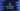# C++ program to find the last character of a string## Introduction :

In this tutorial, we will learn how to find the last character of a string using C++. Our program will get the string from the user and print out the last character of that string. I will show two different ways to solve this problem in this post.

### Method 1: Using index to get the last character of a string in C++ :

We can access the characters of a string using indices. Index starts from 0. The first character index is 0, second character index is 1 etc. If we get the last character index, we can access this character using that index. Thankfully, C++ provides one method called length() for string that returns the length of that string. The last character index of a string is its length - 1. For example, for the string ‘Hello’, its size is 5 and the index of last character is 4. Let me show you the full C++ program :

``````#include <iostream>
#include <string>
using namespace std;

int main()
{
string input_string;

cout << "Enter a string :" << endl;
getline(cin, input_string);

cout << input_string[input_string.length() - 1] << endl;

return 0;
}``````
• In this example, inputstring_ variable is used to hold the user input string.
• We are reading the string using the getline method and storing that value in the variable inputstring_
• inputstring.length()_ method returns the length of the string and we are getting the last character of that string using that index.

Sample output of this program :

``````Enter a string :
hello worlD
D

Enter a string :
hello
o``````

### Method 2: Get the last character using back() in C++ :

back() method is defined in the string class. It returns the last character of a string. For example :

``````#include <iostream>
#include <string>
using namespace std;

int main()
{
string input_string;

cout << "Enter a string :" << endl;
getline(cin, input_string);

cout << input_string.back() << endl;

return 0;
}``````

It will give similar output as the above program. You can use any one of these methods to find the last character in C++.